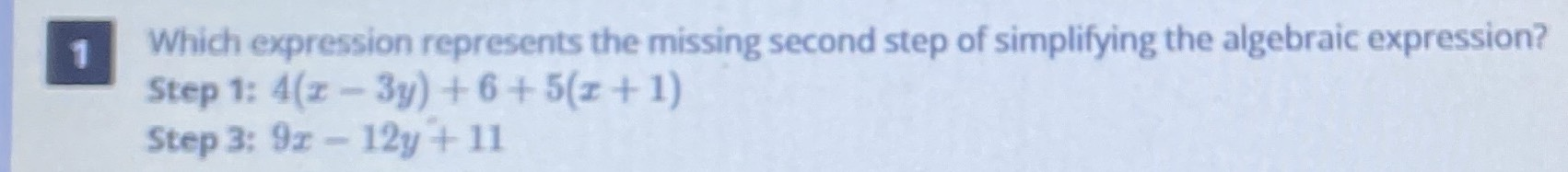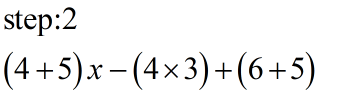### ¿Todavía tienes preguntas de matemáticas?

Pregunte a nuestros tutores expertos
Algebra
Pregunta1 Which expression represents the missing second step of simplifying the algebraic expression?

Step 1: $$4 ( x - 3 y ) + 6 + 5 ( x + 1 )$$

Step 3: $$9 x - 12 y + 11$$